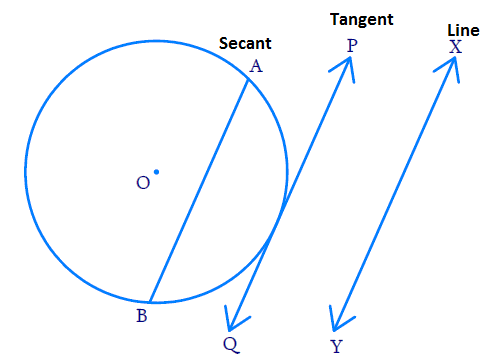In the verge of coronavirus pandemic, we are providing FREE access to our entire Online Curriculum to ensure Learning Doesn't STOP!

# Ex.10.1 Q4. Circles Solution - NCERT Maths Class 10

Go back to  'Ex.10.1'

## Question

Draw a circle and two lines parallel to a given line such that one is a tangent and the other, a secant to the circle.

Video Solution
Circles
Ex 10.1 | Question 4

## Text Solution

What is Known?

(i) To draw a circle

(ii) Draw one tangent and one secant to the circle parallel  to the given line.

What is Unknown?

To draw a circle as per known details.

Steps:$$XY$$ is the given line.

$$AB$$ is the secant parallel to $$XY,$$ $${AB}\parallel {XY}$$

$$AQ$$ is the tangent parallel to $$XY,$$ $${PQ}\parallel {XY}$$

Learn from the best math teachers and top your exams

• Live one on one classroom and doubt clearing
• Practice worksheets in and after class for conceptual clarity
• Personalized curriculum to keep up with school